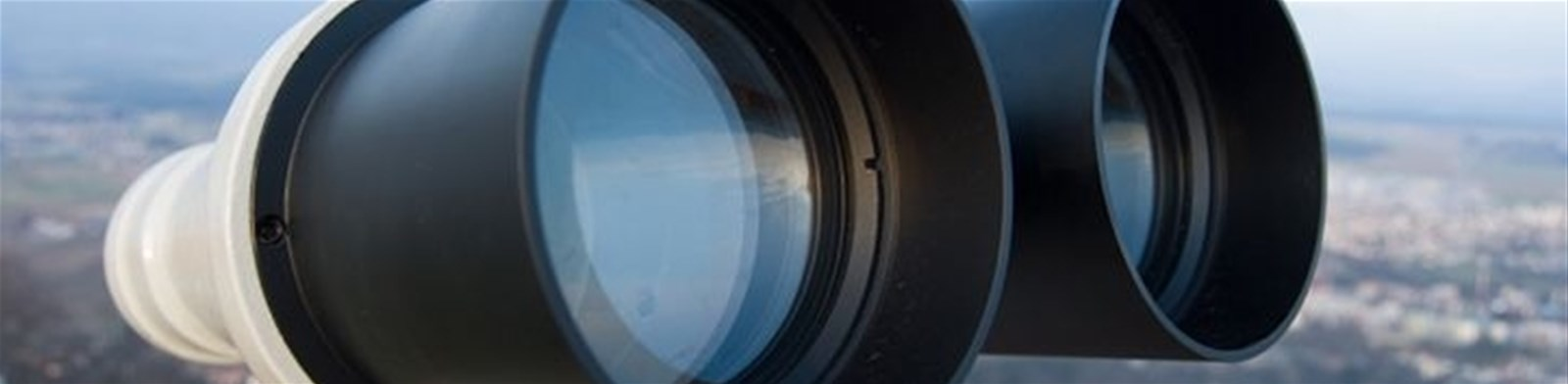Understanding optics...

# Twilight Factor

Twilight factor is a theoretical estimation of how much detail can be seen in low light and is estimated by first multiplying the magnification by the objective lens size, and then taking the square root of that product.

So twilight factor can increase with an increase of either magnification and objective lens size or both. The twilight factor can also remain the same if the magnification is doubled but the objective size is cut in half (and vice versa). Generally the higher the twilight number value the better...

So for example:

30x80 binoculars would be 30 x 80 = 2400. The square root of 2400 = 48.98 value for twilight factor.

10x50 binoculars would be 10 x 50 = 500. The square root of 500 = 22.36 value for twilight factor.

Therefore the 30x80 have a better twilight factor than the 10x50 binoculars

HDC DF Range
30 X 80 = 48.98 twilight factor.
25 X 80 = 44.72 twilight factor.
20 X 80 = 40.00 twilight factor.

HDC SC Range
20 X 60 = 34.64 twilight factor.
10 X 60 = 24.49 twilight factor.
10 X 50 = 22.36 twilight factor.

HDC HS Range

35 X 60 = 45.82 twilight factor.
20 X 60 = 34.64 twilight factor.
10 X 50 = 22.36 twilight factor.

- What do the numbers on my scope mean?

- What is magnification?

- What is the field of view

- What is an objective lens?

- What is eye relief?

- What is the exit pupil?

- Twilight Factor

- Relative Brightness